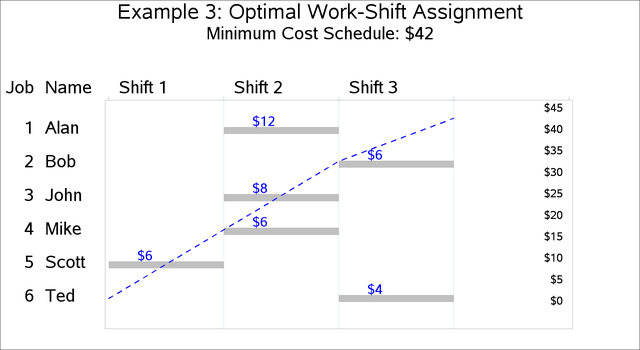### Example 3.3 Work-Shift Scheduling Problem

This example illustrates the use of the GCC constraint in finding a feasible solution to a work-shift scheduling problem and then using the element constraint to incorporate cost information in order to find a minimum cost schedule.

Six workers (Alan, Bob, John, Mike, Scott, and Ted) are to be assigned to three working shifts. The first shift needs at least one and at most four people; the second shift needs at least two and at most three people; and the third shift needs exactly two people. Alan does not work on the first shift; Bob works only on the third shift. The others can work any shift. The objective is to find a feasible assignment for this problem.

You can model the minimum and maximum shift requirements with a GCC constraint and formulate the problem as a standard CSP. The variables W1–W6 identify the shift to be assigned to each of the six workers: Alan, Bob, John, Mike, Scott, and Ted.

proc clp out=clpout;
/*  Six workers (Alan, Bob, John, Mike, Scott and Ted)
are to be assigned to 3 working shifts.            */
var (W1-W6) = [1,3];

/* The first shift needs at least 1 and at most 4 people;
the second shift needs at least 2 and at most 3 people;
and the third shift needs exactly 2 people. */
gcc (W1-W6) = ( ( 1, 1, 4) ( 2, 2, 3) ( 3, 2, 2) );

/* Alan doesn't work on the first shift. */
lincon W1 <> 1;

/* Bob works only on the third shift. */
lincon W2 = 3;
run;



The resulting assignment is shown in Output 3.3.1.

Output 3.3.1: Solution to Work-Shift Scheduling Problem

 Solution to Work-Shift Scheduling Problem

Obs W1 W2 W3 W4 W5 W6
1 2 3 1 1 2 3

A Gantt chart of the corresponding schedule is displayed in Output 3.3.2.

Output 3.3.2: Work-Shift ScheduleNow suppose that every work-shift assignment has a cost associated with it and that the objective of interest is to determine the schedule with minimum cost.

The costs of assigning the workers to the different shifts are given in Table 3.9. A dash - in position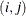indicates that workercannot work on shift.

Table 3.9: Costs of Assigning Workers to Shifts

 Shift 1 Shift 2 Shift 3 Alan - 12 10 Bob - - 6 John 16 8 12 Mike 10 6 8 Scott 6 6 8 Ted 12 4 4

Based on the cost structure in Table 3.9, the schedule derived previously has a cost of 54. The objective now is to determine the optimal schedule—one that results in the minimum cost.

Let the variable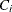represent the cost of assigning workerto a shift. This variable is shift-dependent and is given a high value (for example, 100) if the worker cannot be assigned to a shift. The costs can also be interpreted as preferences if desired. You can use an element constraint to associate the costwith the shift assignment for each worker. For example,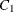, the cost of assigning Alan to a shift, can be determined by the constraint ELEMENT(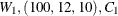).

By adding a linear constraint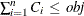, you can limit the solutions to feasible schedules that cost no more than obj.

You can then create a SAS macro %CALLCLP with obj as a parameter that can be called iteratively from a search routine to find an optimal solution. The SAS macro %MINCOST(lb,ub) uses a bisection search to find the minimum cost schedule among all schedules that cost between lb and ub. Although a value of ub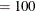is used in this example, it would suffice to use ub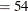, the cost of the feasible schedule determined earlier.

%macro callclp(obj);
%put The objective value is: &obj..;
proc clp out=clpout;
/* Six workers (Alan, Bob, John, Mike, Scott and Ted)
are to be assigned to 3 working shifts.  */
var (W1-W6) = [1,3];
var (C1-C6) = [1,100];

/* The first shift needs at least 1 and at most 4 people;
the second shift needs at least 2 and at most 3 people;
and the third shift needs exactly 2 people. */
gcc (W1-W6) = ( ( 1, 1, 4) ( 2, 2, 3) ( 3, 2, 2) );

/* Alan doesn't work on the first shift. */
lincon W1 <> 1;

/* Bob works only on the third shift. */
lincon W2 = 3;


      /* Specify the costs of assigning the workers to the shifts.
Use 100 (a large number) to indicate an assignment
that is not possible.*/
element (W1, (100,  12, 10), C1);
element (W2, (100, 100,  6), C2);
element (W3, ( 16,   8, 12), C3);
element (W4, ( 10,   6,  8), C4);
element (W5, (  6,   6,  8), C5);
element (W6, ( 12,   4,  4), C6);

/* The total cost should be no more than the given objective value. */
lincon C1 + C2 + C3 + C4 + C5 + C6 <= &obj;
run;

/* when a solution is found,                      */
/* &_ORCLP_ contains the string SOLUTIONS_FOUND=1   */
%if %index(&_ORCLP_,  SOLUTIONS_FOUND=1) %then %let clpreturn=SUCCESSFUL;
%mend;

/* Bisection search method to determine the optimal objective value  */
%macro mincost(lb, ub);
%do %while (&lb<&ub-1);
%put Currently lb=&lb, ub=&ub..;
%let newobj=%eval((&lb+&ub)/2);
%let clpreturn=NOTFOUND;
%callclp(&newobj);
%if &clpreturn=SUCCESSFUL %then %let ub=&newobj;
%else %let lb=&newobj;
%end;

%callclp(&ub);

%put Minimum possible objective value within given range is &ub.;
%put Any value less than &ub makes the problem infeasible. ;

proc print;
run;
%mend;

/* Find the minimum objective value between 1 and 100. */
%mincost(lb=1, ub=100);



The cost of the optimal schedule, which corresponds to the solution shown in the following output, is 40.

 Solution to Optimal Work-Shift Scheduling Problem

Obs W1 W2 W3 W4 W5 W6 C1 C2 C3 C4 C5 C6
1 2 3 2 2 1 3 12 6 8 6 6 4

The minimum cost schedule is displayed in the Gantt chart in Output 3.3.3.

Output 3.3.3: Work-Shift Schedule with Minimum Cost